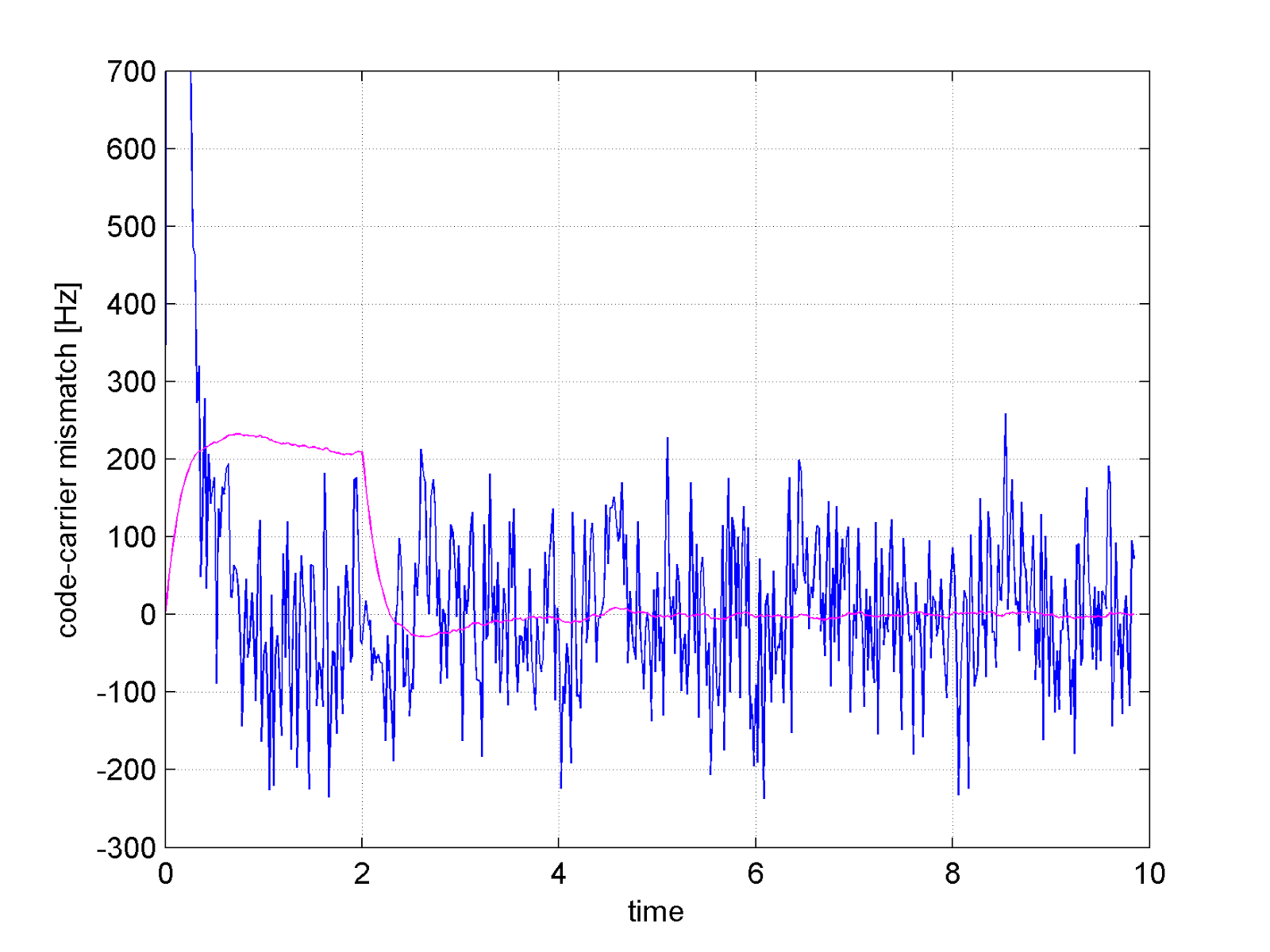## Monday, May 12, 2014

### GNSS carrier phase, RTLSDR, and fractional PLLs (the necessary evil)

A mandatory principle when processing GNSS -in order to have high accuracy carrier phase- is to have a well defined frequency planning. This entails knowing precisely how the Local Oscillator (LO) frequency is generated.
With RTL-SDR it is not a trivial task given that both R820T and RTL2832U use fractional Phase Locked Loops (PLLs) in order to derive respectively the high-side mixing frequency and the Digital Down Conversion (DDC) carrier.
I guess most people use RTL-SDR with a 50ppm crystal so the kind of inaccuracies I am going to describe are buried under the crystal inaccuracy ..within reason.

Let us start from the common call

&gt; rtl_sdr -f 1575420000

This means "set to 1575.42 MHz" but what is hidden is:
1) R820T, set to 1575.42e6 + your IF
2) RTL2832U, downconvert the R820T IF to baseband
.. there are approximations everywhere.

Now, the R820T has a 16 bit fractional PLL register meaning that it can only set to frequencies multiple of 439.45 Hz (exactly).
Instead, the RTL2832U has a 22 bit fractional PLL register meaning that is can recover IFs in steps of 6.8665 Hz (exactly).
Of course, nor 1575.42e6, nor 3.57e6 are exact multiples of either frequency so one always ends up with a mismatch between what he/she thinks he has set, and what really ends up with. Most of the times, this is fine. For GNSS it is not since carrier is accumulated over long intervals and even a few tenths of Hz will make it diverge from the truth.
So I went down the route of characterising the necessary evil of fractional PLLs.

The first test I did was to set the tuner to 1575421875, which leads to a -1875 Hz center frequency but is nicely represented in 16 bits using a 28.8 MHz reference (remember the R820T). In fact, 54 + 0.7021484375 = 54 + /2^16. ..ok well actually it fits on 10 :)

Here I found a small bug in the driver  and replaced the following messy (IMHO) code:

/* sdm calculator */
while (vco_fra > 1) {
if (vco_fra > (2 * pll_ref_khz / n_sdm)) {
sdm = sdm + 32768 / (n_sdm / 2);
vco_fra = vco_fra - 2 * pll_ref_khz / n_sdm;
if (n_sdm >= 0x8000)
break;
}
n_sdm <<= 1;
}

with

mysdm = (((vco_freq<<16)+pll_ref)/(2*pll_ref)) & 0xFFFF;

Then I modified the IF of the R820T from 3.57 MHz to 3.6 MHz, as it is only a few kHz away and it is nicely represented on 16 bit  ..ok well it actually fits in 3 :)
Modifying the IF also impacted the RTL2832U fractional register of course.
I still had a significant error (about 115 Hz) which I could measure comparing the scaled code rate and the carrier rate (which should be proportional of a factor 1540).
After a long time wondering what could be happening, I decided to start tweaking the bits of the R820T.
One in particular called PLL dithering seemed suspicious. Disabling it kind of doubled the error to about 220Hz. Sad.. but I did recall now the resolution of the tuner (439.45 Hz) and guessed that there is a hidden 17th bit which toggles randomly when "dithering" and is instead fixed to 1 when "not dithering". A couple of references which could explain why are here:Figure 1: Code-carrier mismatch when tracking a satellite with RTL-SDR